Computational Method

The building blocks of the analysis are the sums of squares for the dependent variables for each classification variable within the factors that precede it in the model, corrected for the factors that follow it. For example, for a two-factor nested design, PROC NESTED computes the following sums of squares: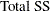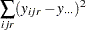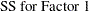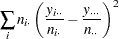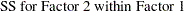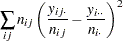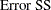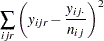where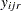is theth replication,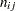is the number of replications at levelof the first factor and levelof the second, and a dot as a subscript indicates summation over the corresponding index. If there is more than one dependent variable, PROC NESTED also computes the corresponding sums of crossproducts for each pair. The expected value of the sum of squares for a given classification factor is a linear combination of the variance components corresponding to this factor and to the factors that are nested within it. For each factor, the coefficients of this linear combination are computed. (The efficiency of PROC NESTED is partly due to the fact that these various sums can be accumulated with just one pass through the data, assuming that the data have been sorted by the classification variables.) Finally, estimates of the variance components are derived as the solution to the set of linear equations that arise from equating the mean squares to their expected values.